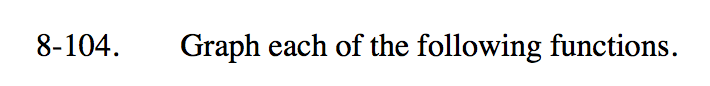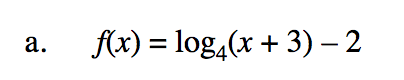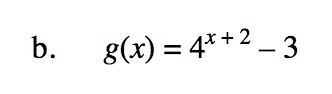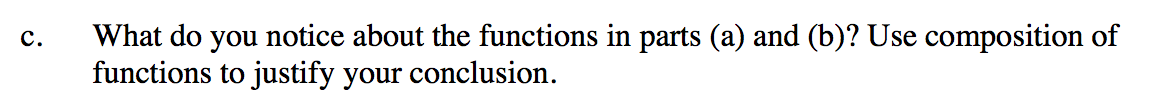### Home > PC3 > Chapter 8 > Lesson 8.3.2 > Problem8-104

8-104.
1. Graph each of the following functions. Homework Help ✎

1. f(x) = log4(x + 3) – 2

2. g(x) = 4x + 2 – 3

3. What do you notice about the functions in parts (a) and (b)? Use composition of functions to justify your conclusion.Rewrite the equation in exponential form.
Then make a table, choosing the y-values and then calculating the x-values.

$y+2=\log_4(x+3)$This is exponential growth. The curve is shifted 2 units left and 3 units down.